# D Flip Flop Explained in Detail

Flip – flops are one of the most fundamental electronic components. These are used as one-bit storage elements, clock dividers and also we can make counters, shift registers, and storing registers by connecting the flip flops in particular sequences. These flip flops use feedback concept to create sequential logic where the previous state affect future states (unlike combinational circuit).

The common types of flip flops are as follows:

The D in the D flip flop represents the data (generation, processing, or storing) in the form of states. The two states are binary, 0 (Low) and 1 (High), set or reset, positive or non-positive.

So, let us discuss the latches (Flip flop) first. The latches are as Bistable Multivibrator as two stable states. And of course, these circuits are triggered by Low or High signals.

Well, you must get a question in mind!!

Why do we need D flip flops? Think!

The answer is pretty much simple, though. This is because of the disadvantage of the basic SR NAND gate Bistable circuit. It gives an invalid state when both set and reset are ‘0’ (active Low).

## D LatchFig:Logic Symbol

Looking at the truth table of the SR latch we can realize that when both inputs are the same, the output either does not change or it is invalid (Inputs = 00, no change and inputs = 11, invalid). In many of the practical applications, these input conditions are not required. These inputs condition can be avoided by making them complement of each other. This modified version of SR latch is known as D latch.Fig:D latch

The above figure shows the D latch. The NAND gates 1, 2, 3, and 4 form the basic SR latch with enable input. The use of the fifth NAND gate is to provide the complemented inputs.

As shown in fig, D input goes directly to the S input, and its complement is applied to the R input, through gate 5. Thus, only two input conditions exists, either S = 0 and R = 1 or S = 1 and R = 0. The truth table for D latch is as shown in the below table.

## Truth Table for D latch

 EN D Qn Qn+1 Stable 1 0 X 0 Reset 1 1 X 1 Set 0 X X Qn No change (NC)

As shown in the truth table, the Q output follows the D input. For this reason, D latch is sometimes called a transparent latch.

Looking at the truth table for D latch with enable input and simplifying Qn+1 function by k-map we get the characteristic equation for D latch with enable input as

Qn+1 = EN * D + (EN)’ * Qn

## Clocked D Flip-Flop

Like in D latch, in D flip-flop also, the basic SR flip flop is used with complemented inputs. The D flip flop is similar to D latch except clock pulse followed by edge detector is used instead of enable input. Such an edge-triggered D flip flop can be of two types:

• Positive edge-triggered D flip flop
• Negative edge-triggered D flip flop

### Positive Edge Triggered D flip flop

It consists of a gated D latch and a positive edge detector circuit. As shown in the truth table below, the circuit output responds to the D input only at the positive edges of the clock pulse. At any other instants of time, the D flip flop will not respond to the changes in input.

 CP D Qn+1 I 0 0 I 1 1 0 X Qn

Looking at the truth table for the D flip flop we can realize that Qn+1 function follows D input at the positive-going edges of the clock pulses. Hence the characteristic equation for D flip flop is  Qn+1 = D. However, the output Qn+1 is delayed by one clock period. Thus, D flip flop is also known as delay flip – flop.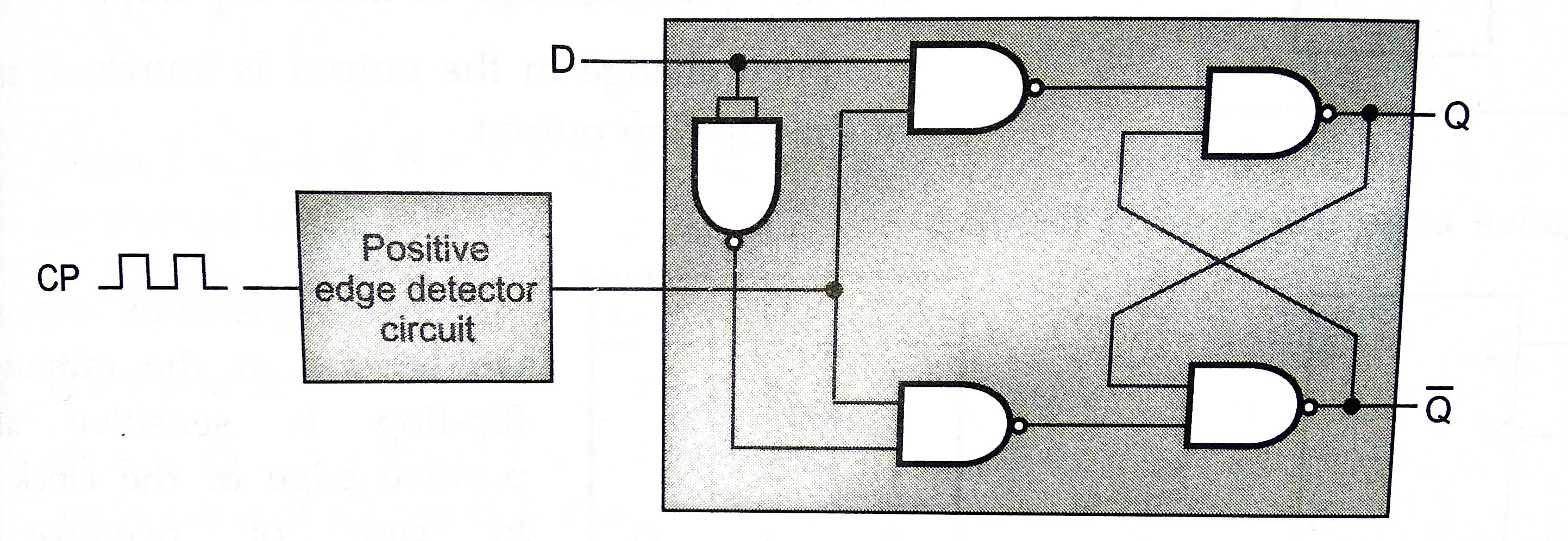Fig: Positive edge-triggered D flip flopFig: Input and output waveforms of clocked D flip flop

If we connect the Q’ output of D flip flop to its D input, the output of D flip flop will change either from 0 to 1 or from 1 to 0 at every positive edge of the D flip flop. Such a change in the output is known as toggling of the flip flop output.

### Negative Edge Triggered D Flip Flop

In the above explanation, we have seen the output of D flip flop is sensitive at the positive edge of the clock input. In the case of negative edge triggering, the output is sensitive at the negative edge of the clock input.

 CP D Qn+1 I 0 0 I 1 1 0 X Qn

The above truth table is for negative edge triggered D flip flop. Also, the input and output waveforms for negative edge triggered flip flop is as shown below: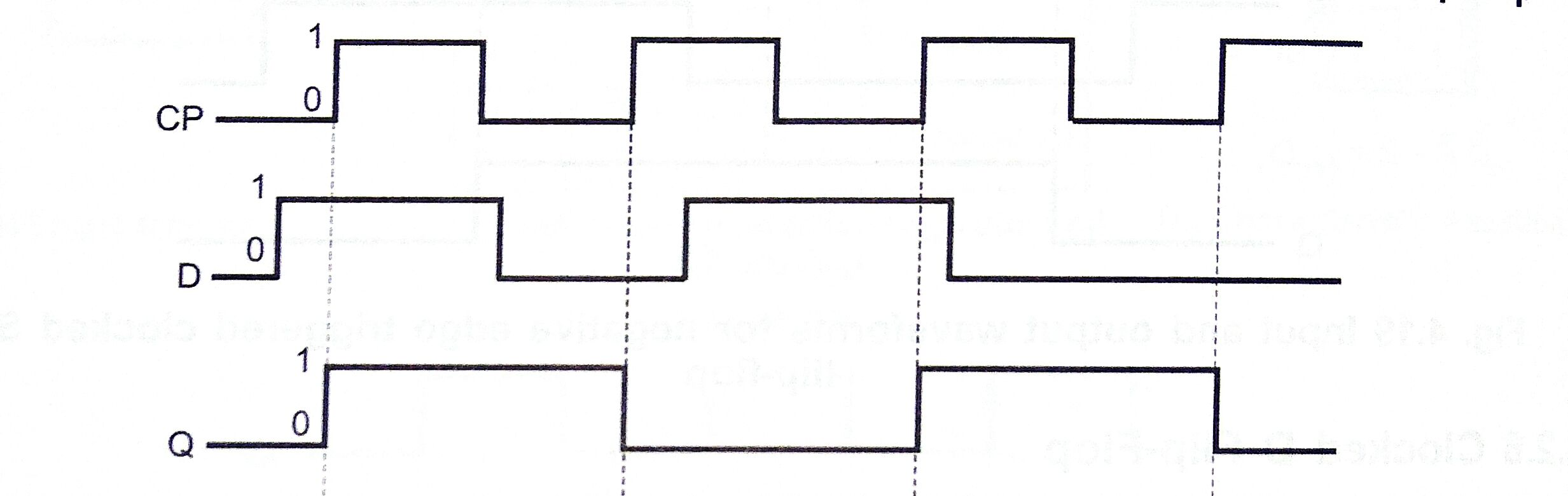Fig: Input and output waveforms of negative edge D flip flop

## D Flip Flop Excitation Table

 Qn Qn+1 D 0 0 0 0 1 1 1 0 0 1 1 1

Table: D Excitation Table

 D Qn+1 0 0 1 1

Table: D Truth Table

The above tables show the excitation table and truth table for D flip flop, respectively. In D flip flop, the next state is independent of the present state and is always equal to the D input. Therefore, D must be 0 if Qn+1 has to be 0, and 1 if Qn+1 has to be 1, regardless of the value of Qn

Now, if we look for an improved version of this D flip flop then, of course, we can achieve it. We will add a second S R flip flop to its output. Let’s see how it improves performance.

## The Master-Slave D Flip Flop

As said above, a second SR flip flop will be added to the output of the basic D type flip flop. It activates on the complementary clock signal to produce the “Master-Slave D flip flop”. At the first stage (clock signal going from Low to High) the Master latches the input condition at D whereas the output stage is deactivated.

At the second stage (clock signal going from High to Low), the slave stage activates. Slave latches on to the output from the first master circuit. This makes the output stage trigger on the negative edge of the clock pulse. This Master-Slave D flip flop is constructed by cascading the two latches having opposite phases. This is shown below.

### The Master-Slave D Flip Flop Circuit

As we are seeing in the figure, Master D flip flop gets the data from D input on the leading edge of the clock pulse (signal going from Low to High). Therefore, the master is ‘ON’ now. Similarly, on the trailing edge of the clock pulse (signal from High to Low), the slave flip flop loads data, i.e., the slave gets ‘ON’.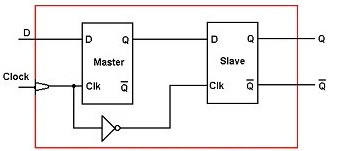Fig: Master-slave D flip flop

Thus, there will always be one flip flop of the master or slave which would be ON and the other would be OFF at one time. This will make output Q acquire the value of D only when one full complete pulse (0-1-0) is applied at the clock input.

## Applications of D Flip Flop

Now, after we know how this flip flop works, we must know that what we can do with this.

Don’t you think that whatever we study has some application else why would we study all these?

There are various applications of D flip flops. Let us explore some which are listed below:

### D type Flip Flop for Frequency Division

This is one of the main use of D flip flop. If we connect the Q’ output of the D type flip flop directly to the D input making the closed-loop feedback. The successive clock pulses would make the bistable toggle one time for every two clock cycles.

Data latch is used as a binary divider or a frequency divider. It produces a divide by 2 counter circuits, i.e., the output frequency will have half the frequency that of the clock pulses.

One more interesting thing that happens here is that we can construct a T type flip flop which can be used as a divide by 2 circuits in binary counter.

### Divide-by-2 Counter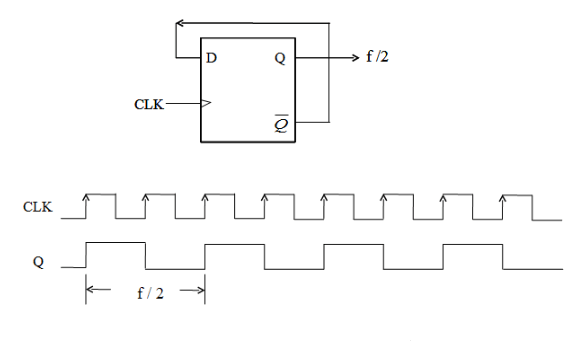Fig: Divide-by-2 counter

From the above frequency waveform, by connecting (feedback) the output Q’ to the input terminal D, the output pulses at Q has a frequency which is exactly half to that of the input clock frequency (fin). Therefore, we can say that the circuit is producing frequency division. It is dividing the frequency by a factor of 2, once for every two clock cycles.

### Transparent Data Latch

The data latch is a useful device in computer and electronic circuits. It is designed in such a way to have a very high impedance at both the outputs Q and its inverse Q’. This reduces the impedance effect on the connecting circuit. For example, when it is used as a buffer, bi-directional bus driver, a buffer, or even a display driver.

Now, it is obvious that a one-bit transparent latch is not useful practically. In fact commercial chips incorporate 4, 8, 10, 16, or 32 individual data latches into one single IC package (example: 74LS373 Octal D type transparent latch).

Let us understand the above explanation in an easier way. For instance, consider we have 8 individual data latches. Therefore, when the clock pulse is High (Logic 1) then the output Q will follow the D input. So, whatever we give at D, comes as output from Q, thus it acts as a buffer.

#### 4 – bit Data Latch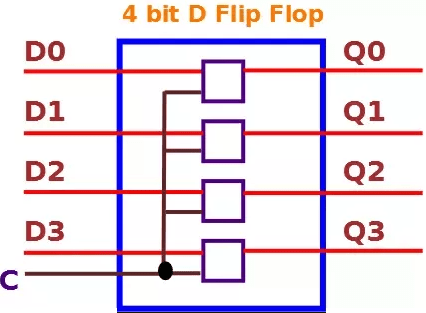Fig: 4-bit D latch

It is the same as explained above. The individual latches will be clubbed together to form the 4-bit data latch. Therefore, as we give data at individual D inputs we can parallelly take the same output from Q.

### Some Applications of Flip Flops

• It is used as delay elements.
• Flip flops are used as memory elements.
• It eliminates key debounce.
• Flip flops are the basic building block in sequential circuits such as registers and counters.

AJAY DHEERAJ
(Technical Content Developer)

•
•
•
•
•
•